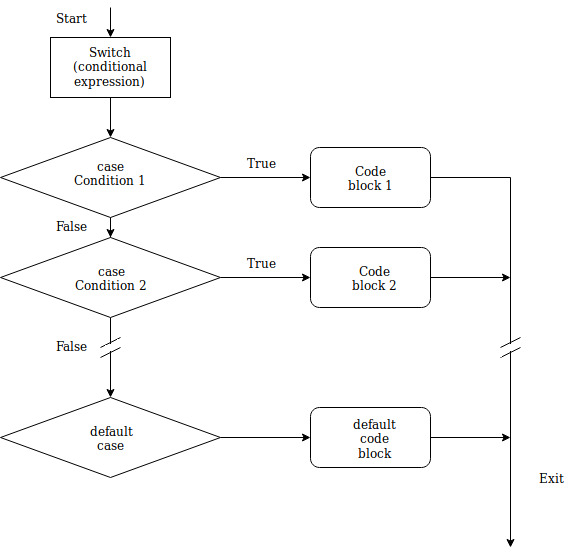×

Search anything:

# Switch Case in Java

#### Software Engineering Java switch caseGet this book -> Problems on Array: For Interviews and Competitive Programming

A switch statement in Java allows an expression to be tested for equality against a list of values. Each value with which the expression in switch is tested against, is called a case.

Basically, a Switch works with byte, short, char and int primitive data types. Beginning with Java SE 7, it also works with enumerated types (Enums), the String class and Wrapper classes.

• What is the syntax of switch case?
• What is the control flow diagram in switch case?
• What is the role of break in switch case?
• What is default statement in switch case?
• Can switch case be nested?
• What are the rules for switch case?
• Can we use Strings in switch case?

### Syntax of `switch case` in Java

Following is the syntax of switch case in Java:

``````switch(expression){
case value1 : //codes to be executed when expression equals value1
break; //break is optional

case value2 : //codes to be executed when expression equals value2
break;

default : //codes to be executed when none of the above execute.
break;
}
``````

### Control Flow diagram of switch case### What is the role of Break statement in Switch case?

Break statement terminates the enclosing switch statement. The syntax is correct without them but they are necessary because in their absence, the statements in switch blocks fall through, i.e execution will continue on into the next case, regardless of its expression, until the switch block ends or a break statement is encountered.

Example :

``````class Opengenus
{
public static void main (String[] args) throws java.lang.Exception
{
int n = 2;
System.out.println("Output without break statement:");
switch(n){
case 1: System.out.println("The value of n is 1");
case 2: System.out.println("The value of n is 2");
case 3: System.out.println("The value of n is 3");
default: System.out.println("The value of n is something else.");
}
System.out.println("\nOutput with break statement:");
switch(n){
case 1: System.out.println("The value of n is 1");
break;
case 2: System.out.println("The value of n is 2");
break;
case 3: System.out.println("The value of n is 3");
break;
default: System.out.println("The value of n is something else.");
break;
}
}
}
``````

The output of the following piece of code is:

``````Output without break statement:
The value of n is 2
The value of n is 3
The value of n is something else.

Output with break statement:
The value of n is 2
``````

### What is Default statement in switch case?

The default statement contains the block of code to be executed when none of the case matches the condition.

### Can switch case be nested?

Yes, nested switch case are supported by Java and are widely used as well.

A nested switch is one in which we use a switch as a part of statement of an outer switch.

Example :

``````int a=0, b=1;
switch(a)
{
case 0: switch(b)
{
case 0: System.out.println("a is 0 and b is 0");
break;
case 1: System.out.println("a is 0 and b is 1");
break;
}
}
``````

Output:

``````a is 0 and b is 1
``````

### What are the Rules for Switch case?

1. The expression in case should result in a constant value (you cannot use variables in the expreession), whereas the expression in switch can be comprised of constants and variables.
Also, the expression in case should be of the same data type as that of the expression in switch.

The following expressions are valid with switch:

``````switch(a+b*c)
switch(a+5)
``````

The follwing expressions are valid with case:

``````case (4-3)
case (4-1*2)
``````

The following expressions are invalid with case:

``````case (a+5)
case (a)    //where a is an int variable
``````
1. Duplicate case values are not allowed. For the same reason, you cannot put two default labels.

The following is not valid:

``````switch(n)
{
case 1: //statements to execute
break;
case 1: //statements to execute
}
``````

### Can we use Strings in switch case statements?

In Java SE 7 and later, the support for a String object is also available with switch case. (You can also have simple expressions with concatenation of strings using the operator '+'.)

Example:

``````String s = "ab";
switch(s+'c')
{
case ("a"+"b"): System.out.println("String is ab.");
break;
case "abc": System.out.println("String is abc.");
break;
}
``````

The output is:

``````String is abc.
``````

## Question on Switch Case

Consider the following block of code:

``````int a=8;
switch(a)
{
case ((int)Math.pow(2,3)): System.out.println("First!");
break;
case 8: System.out.println("Second!");
break;
}
``````

#### What will be the output ?

First!
Second!
Compilation error: constant expression required
Compilation error: duplicate case label
The expression with the first case contains a function. The return value from a function is not fixed. Hence, we are getting the 'constant expression required' error.